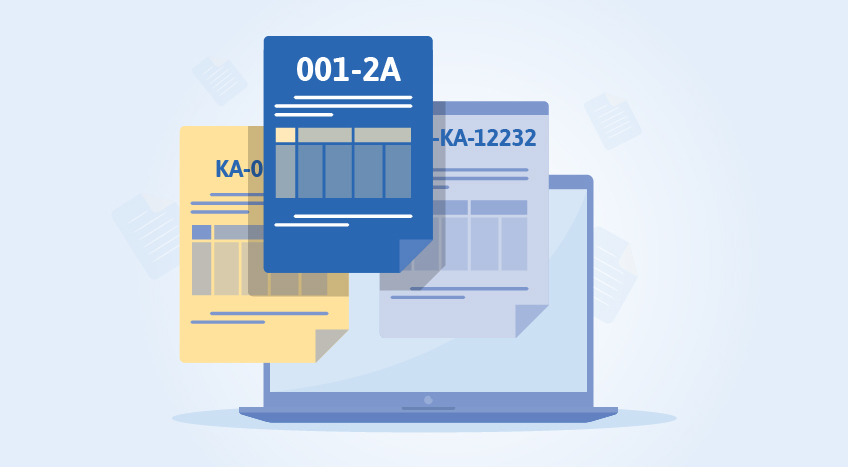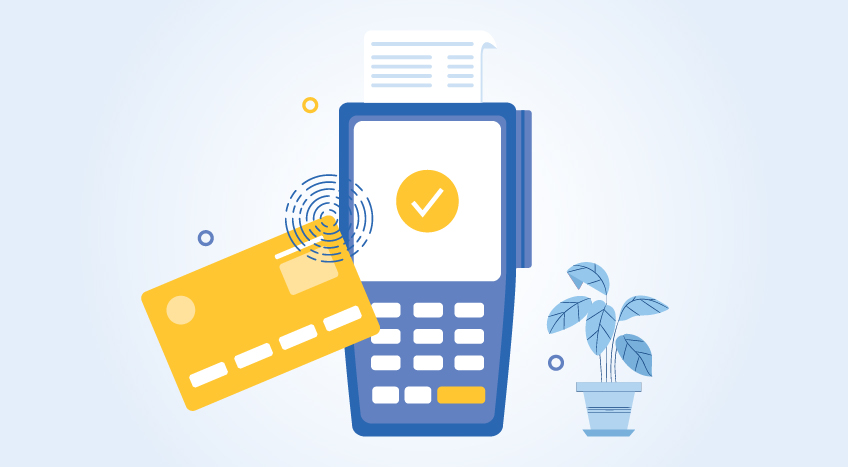# How To Calculate VAT In Tanzania?

## VAT calculation in Tanzania

VAT calculation in Tanzania is based on the taxable value. Let us say that the taxable value is TZS 10,000 and the VAT rate is 18%. This denotes that 18% of TZS 10,000 will be VAT added on top of the taxable value. This is the total that needs to be paid.

Let us calculate the VAT value first.

VAT = VAT rate in percentage x Taxable value

VAT = (18/100) x 10,000

VAT = 1,800

The VAT is TZS 1,800 when the taxable amount is TZS 10,000 and the VAT rate is 18%. The amount that needs to be paid is the VAT value which is TZS 1,800 plus the taxable value which is TZS 10,000. This means the total value to be paid is TZS 11,800.

## VAT inclusive calculation

When a product is VAT inclusive it means that the tax has already been added to the price and the price that you see on the product is what you pay. You do not need to pay VAT separately in such cases because they have already been added to the final price that you will pay the seller. If you wish to calculate the VAT levied on the product and find out the original price of the product then you need to use the following formula:

VAT amount = (Final product price inclusive of VAT) x ( VAT rate / (100 + VAT rate) )

Where;

VAT amount is the value in TZS and it signifies the VAT of the product which was added to the final product price.

The final product price is the VAT-inclusive price that you paid when purchasing it from the seller.

The VAT rate is the TRA specified rate for that product. In Tanzania, Vatable products have a flat VAT rate of 18%.

## Example of VAT inclusive calculation

Let us say that you bought a pair of jeans for TZS 20,000 and this price includes the VAT that has been applied to it. Let us say that the VAT rate is 18%. By using the following formula, we can calculate  the VAT amount:

VAT amount = (Final product price inclusive of VAT) x ( VAT rate / (100 + VAT rate) )

VAT amount = (20,000) x ( 18 / (100 +18) )

VAT amount = (20,000) x (0.1525)

VAT amount = TZS 3,050

This means the price of the jeans before VAT was TZS 16,950. This was computed by subtracting the VAT inclusive final price of the product by the VAT amount. The total VAT amount of TZS 3,050 was applied to the price which gave the final price of TZS 20,000.

## VAT exclusive calculation

When a product is VAT exclusive, it means that the final price of the product does not include VAT. This means VAT is charged separately and added to the bill so you need to pay the total amount which includes the product price and VAT. VAT is charged on a per-product basis and so when the seller gives you a receipt, you can clearly see how much VAT has been applied to each product. By using the VAT exclusive calculation, you can understand the total and final price of the product that includes the VAT by adding the price with the VAT amount giving you the final price. The formula for VAT exclusive calculation is as follows.

Final price of product = VAT amount + VAT exclusive price of the product

Where VAT exclusive price of the product is the  selling price of the product without the VAT and VAT amount is the amount of VAT in TZS to be added to the product and it is calculated using the following formula.

VAT amount = (VAT exclusive price of product) x (Rate of VAT / 100)

Where the rate of VAT is the VAT specified by the TRA according to the goods and services.

## Example of VAT exclusive calculation

The VAT exclusive calculation is better understood with an example. Let us say that you purchased a toy for your child at TZS 30,000 and a VAT of 18% has to be applied to it. This is how you will calculate the final price of the product.

First, calculate the VAT amount;

VAT amount = (VAT exclusive price of product) x (Rate of VAT / 100)

VAT amount = (30,000) x (18 / 100)

VAT amount = 5,400

This means the VAT to be applied to the product at an 18% VAT rate is TZS 5,400.

Now to calculate the final price of the product so that it includes VAT;

Final price of product = VAT amount + VAT exclusive price of the product

Final price of product = 5,400 + 30,000

Final price of produce = 35,400

When VAT is applied to the TZS 30,000 toy, the total that you will need to pay is TZS 35,400.

#### Latest Blogs